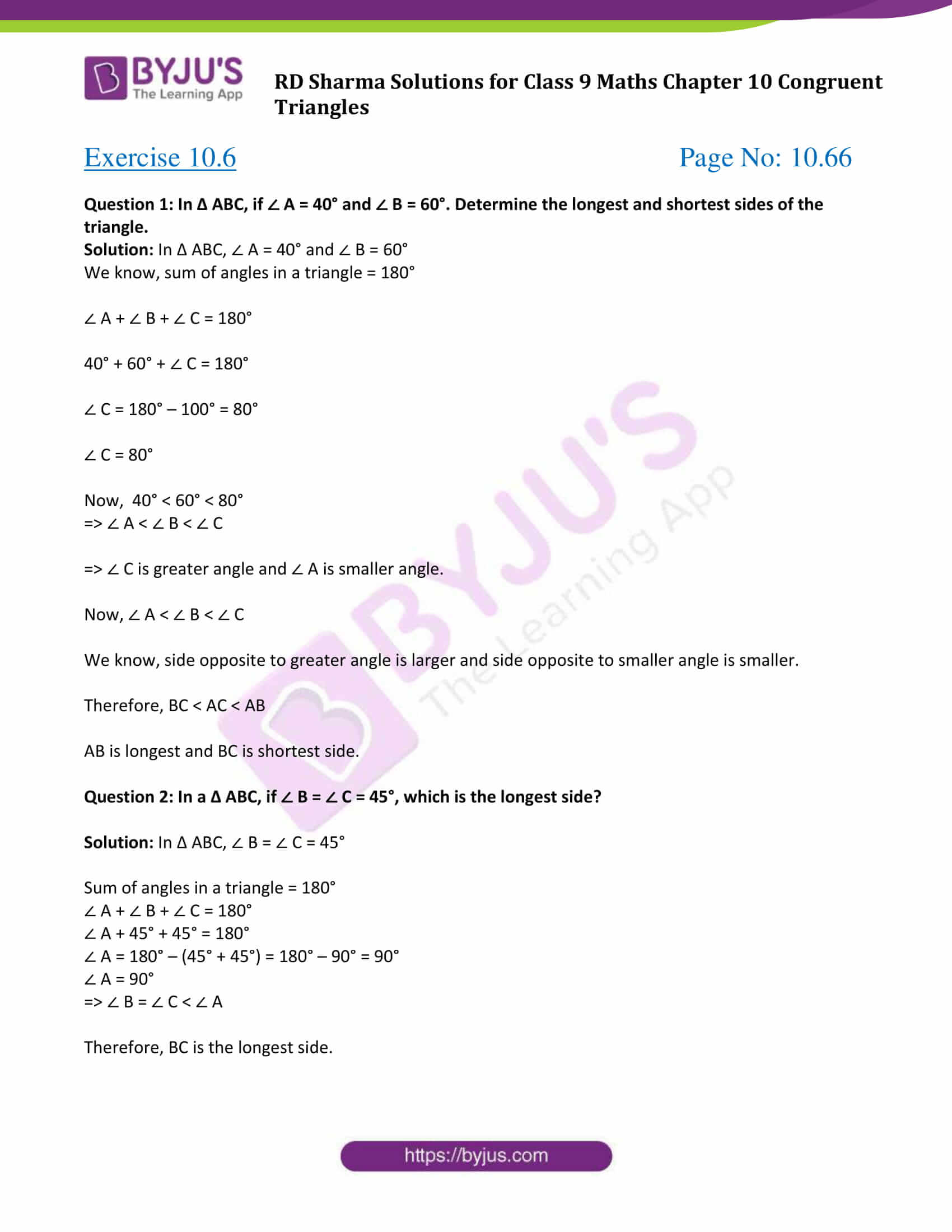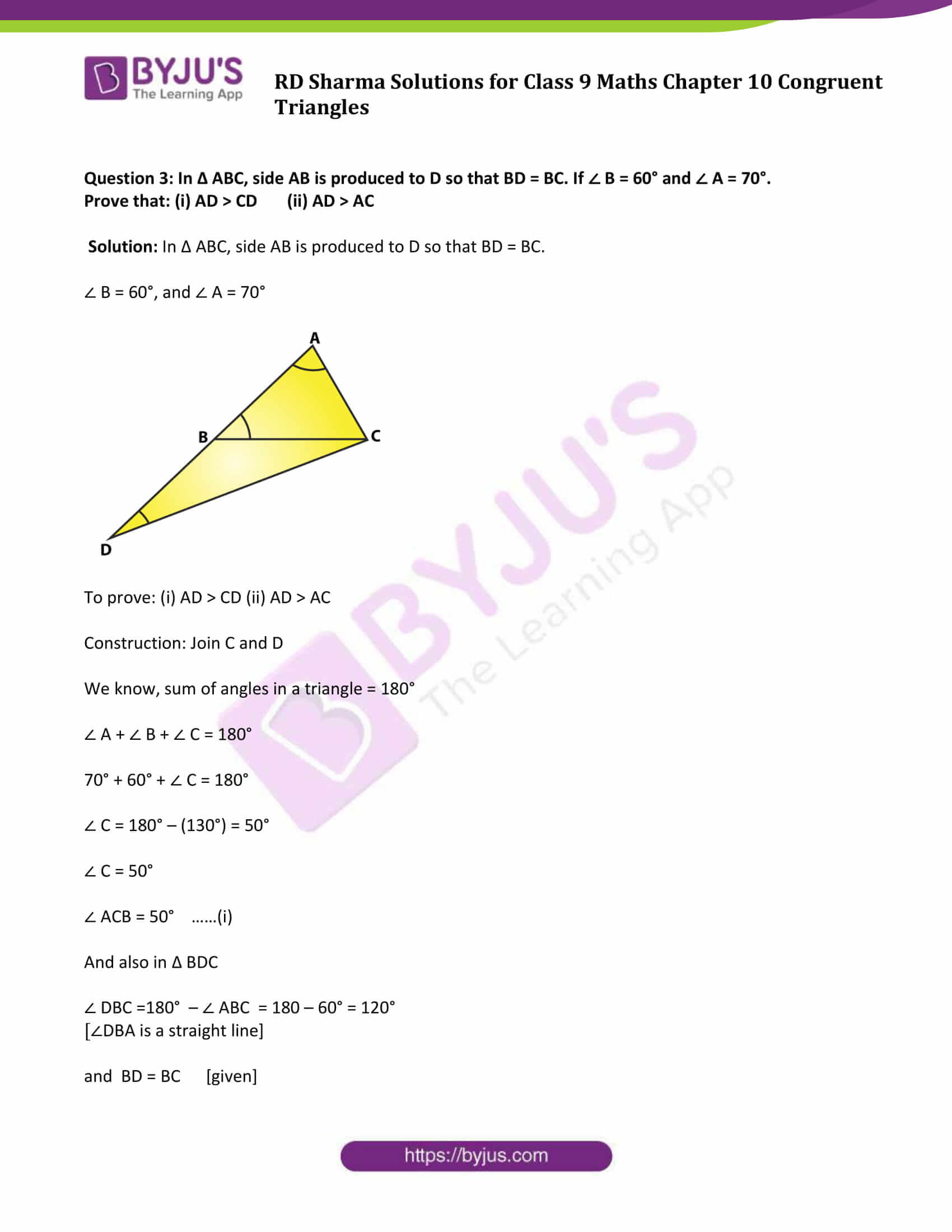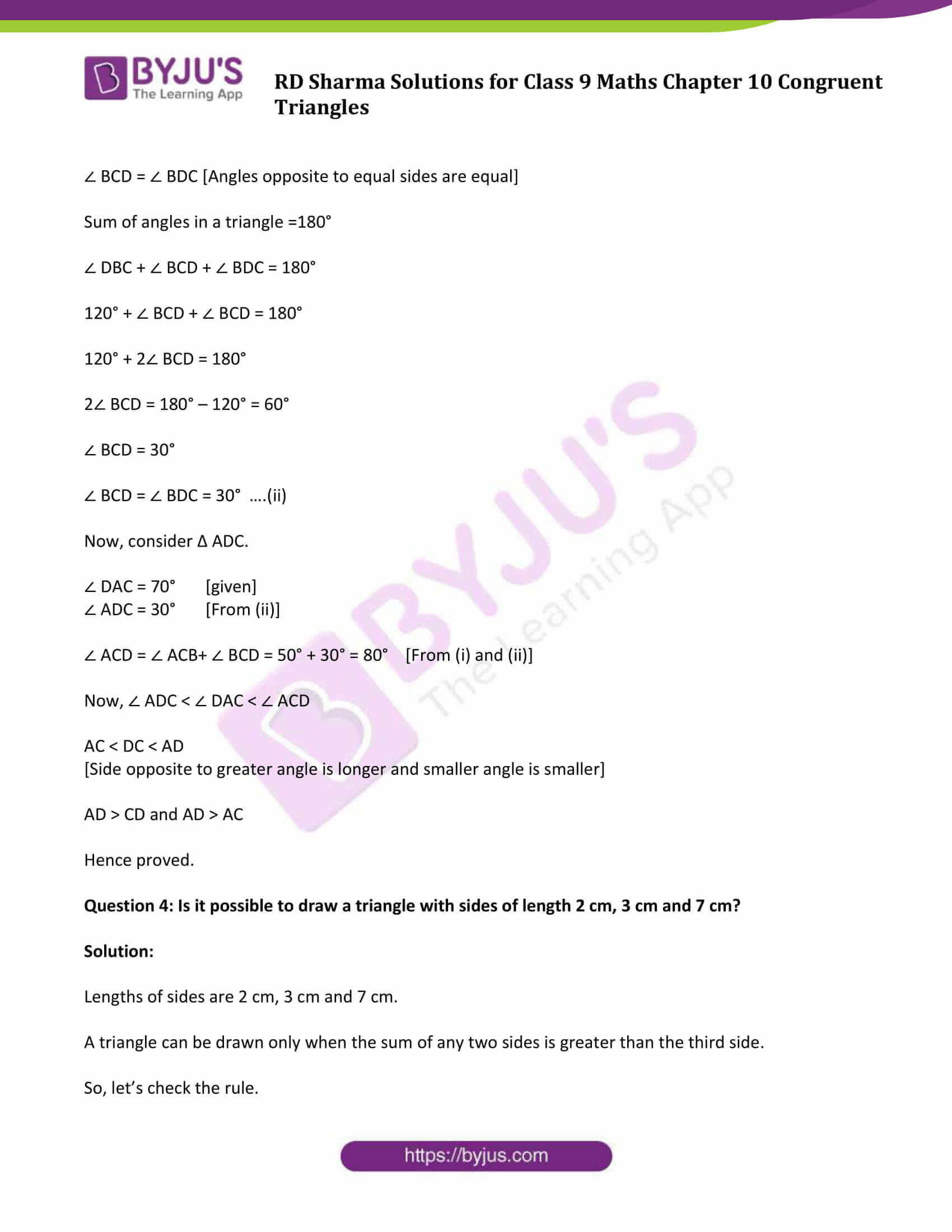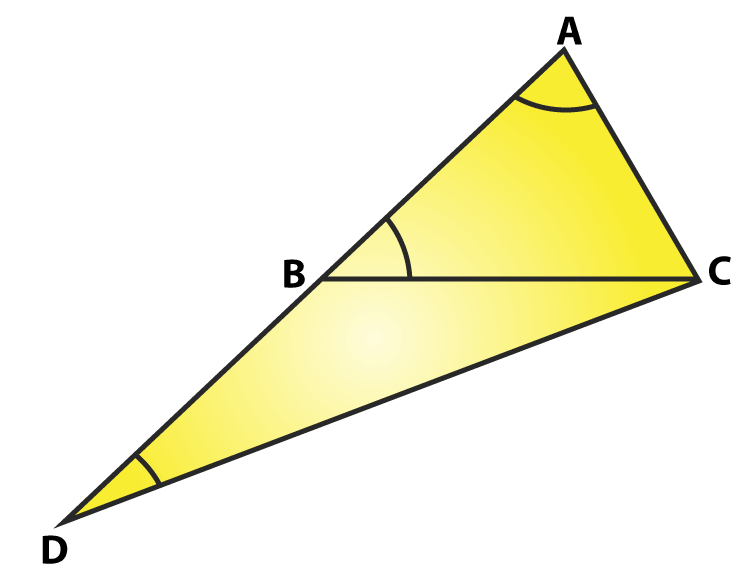# RD Sharma Solutions for Class 9 Maths Chapter 10 Congruent Triangles Exercise 10.6

RD Sharma class 9 Mathematics chapter 10 exercise 10.6 Congruent Triangles solutions are provided here. In this exercise, students can get the detailed RD Sharma class 9 solutions for chapter 10 exercise 10.6 in an easy way to understand. Exercise 10.6 question-answers help students to learn about congruence criterion of right triangles mainly using RHS (Right angle-Hypotenuse-Side) and its related concepts.

## Download PDF of RD Sharma Solutions for Class 9 Maths Chapter 10 Congruent Triangles Exercise 10.6### Access Answers to Maths RD Sharma Solutions for Class 9 Chapter 10 Congruent Triangles Exercise 10.6 Page number 10.66

Question 1: In Δ ABC, if ∠ A = 40° and ∠ B = 60°. Determine the longest and shortest sides of the triangle.

Solution: In Δ ABC, ∠ A = 40° and ∠ B = 60°

We know, sum of angles in a triangle = 180°

∠ A + ∠ B + ∠ C = 180°

40° + 60° + ∠ C = 180°

∠ C = 180° – 100° = 80°

∠ C = 80°

Now, 40° < 60° < 80°

⇒ ∠ A < ∠ B < ∠ C

⇒ ∠ C is greater angle and ∠ A is smaller angle.

Now, ∠ A < ∠ B < ∠ C

We know, side opposite to greater angle is larger and side opposite to smaller angle is smaller.

Therefore, BC < AC < AB

AB is longest and BC is shortest side.

Question 2: In a Δ ABC, if ∠ B = ∠ C = 45°, which is the longest side?

Solution: In Δ ABC, ∠ B = ∠ C = 45°

Sum of angles in a triangle = 180°

∠ A + ∠ B + ∠ C = 180°

∠ A + 45° + 45° = 180°

∠ A = 180° – (45° + 45°) = 180° – 90° = 90°

∠ A = 90°

⇒ ∠ B = ∠ C < ∠ A

Therefore, BC is the longest side.

Question 3: In Δ ABC, side AB is produced to D so that BD = BC. If ∠ B = 60° and ∠ A = 70°.

Solution: In Δ ABC, side AB is produced to D so that BD = BC.

∠ B = 60°, and ∠ A = 70°Construction: Join C and D

We know, sum of angles in a triangle = 180°

∠ A + ∠ B + ∠ C = 180°

70° + 60° + ∠ C = 180°

∠ C = 180° – (130°) = 50°

∠ C = 50°

∠ ACB = 50° ……(i)

And also in Δ BDC

∠ DBC =180° – ∠ ABC = 180 – 60° = 120°

[∠DBA is a straight line]

and BD = BC [given]

∠ BCD = ∠ BDC [Angles opposite to equal sides are equal]

Sum of angles in a triangle =180°

∠ DBC + ∠ BCD + ∠ BDC = 180°

120° + ∠ BCD + ∠ BCD = 180°

120° + 2∠ BCD = 180°

2∠ BCD = 180° – 120° = 60°

∠ BCD = 30°

∠ BCD = ∠ BDC = 30° ….(ii)

∠ DAC = 70° [given]

∠ ADC = 30° [From (ii)]

∠ ACD = ∠ ACB+ ∠ BCD = 50° + 30° = 80° [From (i) and (ii)]

Now, ∠ ADC < ∠ DAC < ∠ ACD

[Side opposite to greater angle is longer and smaller angle is smaller]

Hence proved.

Question 4: Is it possible to draw a triangle with sides of length 2 cm, 3 cm and 7 cm?

Solution:

Lengths of sides are 2 cm, 3 cm and 7 cm.

A triangle can be drawn only when the sum of any two sides is greater than the third side.

So, let’s check the rule.

2 + 3 ≯ 7 or 2 + 3 < 7

2 + 7 > 3

and 3 + 7 > 2

Here 2 + 3 ≯ 7

So, the triangle does not exit.

## RD Sharma Solutions for Class 9 Maths Chapter 10 Congruent Triangles Exercise 10.6

Class 9 Maths Chapter 10 Congruent Triangles Exercise 10.6 is based on the topic- Some inequality Relations in a Triangle.

As we have learnt that if two sides of a triangle are equal to the angles opposite to them are also equal and vice-versa. What happens when two angles of a triangle are unequal? Confused? Do not worry! RD Sharma Solutions chapter 10 will answer all your questions in the form of theorems. Students can download this study material now for free and clear all their doubts.